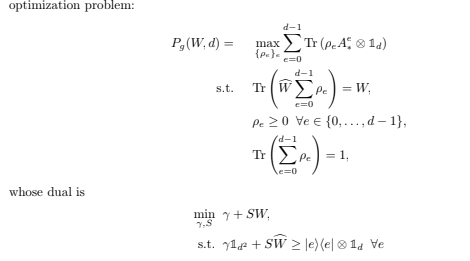# Status Failed: Optimal Value is NaN

Dears,
I have this optimization problem:I am trying to write code for thr dual part. However I have minus G value: I dont understand why.
Could you please kindly look at and let me know?

``````function [G,W,S] = TD_P_inv_Dual(P_exp,B,A_ax,B_by)

d = size(P_exp,1); %dimentions
m = size(P_exp,3); %number of operators
%d = size(B_by,1);
%m = size(B_by,3);
cvx_begin

expression S
W_hat=zeros(d^2,d^2);
for a=1:d
for b=1:d
for x=1:m
for y=1:m
W_hat=W_hat+B(a,b,x,y).*(kron(A_ax(:,:,x,a),B_by(:,:,y,b)));
end
end
end
end
W=sum(sum(sum(sum(B.*P_exp)))); %This is W
J=cvx(0);

for a=1:d
for b =1:d
for x=1:m
for y =1:m
if a ~= b
S = sum(B(a,b,x,y));
J=S*W;
end
end
end
end
end
J=real(J)
minimize(J)

subject to

for e=1:d
(S.*W_hat-kron(squeeze(A_ax(:,:,1,e)),eye(d)))==semidefinite(d^2,d^2); %this part is the following part of eq. 19
end
cvx_end
G=double(S.*W);``````

Your CVX program doesn’t have any variable declarations. I don’t think such a program can do anything useful 9from an optimization or feasibility problem perspective.

1 Like

B is actually a variable which is come from the other code so I am using it directly. Should I declare it again ? and S is an expression whihc is built from B

ahh so sorry I forgat the gamma in the formula I added it and it is ok now
sorry again …

O.k., if an argument to the function is already a CVX function or expression, then it should not be declared again.

Has your difficulty now been resolved?

1 Like

Now the problem has been solved. I mean at least G is non negative and status is solved.
The thing is that I could not find any explanation for gamma so I just ignore that. However When I saw your message I thought that this gamma should be a variable in the code so I added gamma as a variable to code and now it seems ok
Thank you very mych Mark_L_Stone :)